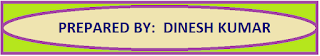### Resource Centre Mathematics

Resource Centre Mathematics Mathematics worksheet, mathematics basic points and formulas, mathematics lesson plan, mathematics multiple choice questions Workplace Dashboard CBSE Syllabus For Session 2023-24 For  :   Classes IX & X    |   Classes XI & XII Watch Videos on Maths Solutions CLASS IX MATHEMATICS FORMULAS &  BASIC CONCEPTS

# Assignments Class 11 Mathematics

Chapter-wise mathematics assignment for class 11 according to the CBSE and DAV Board syllabus. Important and useful extra questions with answer hints to the difficult questions.

CBSE Mathematics is a very good platform for the students and is contain the assignments for the students from 9th to 12th standard.

Here students can find very useful content which is very helpful to handle final examinations effectively.

For better understanding of the topic students should revise NCERT book with all examples and then start solving the chapter-wise assignments.

These assignments cover all the topics and are strictly according to the CBSE syllabus.

With the help of these assignments students can easily achieve the examination level and  can reach at the maximum height.

 Class 11Mathematics  Assignment Case Study Based QuestionChapter 1 Set TheorySolution of NCERT Ch-1 Set TheoryChapter 2 Relations & FunctionsChapter 3 TrigonometryChapter 4 Principal of Mathematical InductionChapter 5 Complex NumbersChapter 6 Linear InequalitiesChapter 7 Permutations & CombinationsChapter 8 Binomial TheoremChapter 9 Sequence and SeriesChapter 10 Straight LinesChapter 11 Conic SectionsChapter 12 Three Dimensional GeometryChapter 13 Limits Chapter 13 DerivativesChapter 14 Mathematical ReasoningsChapter 15 StatisticsChapter 16 Probability

##CBSE Mathematics also contains chapter-wise maths formulas /basic points / definitions / lesson plans useful for the teachers and many other blogs which are useful for all maths loving people.

A platform for the mathematics student is provided where students can fulfill all their study related needs. So click on the link below to enter the RESOURCE CENTRE.